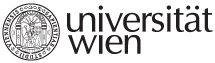Name of course DXE_MIKR Microeconomics Lecturer Leopold Sögner, Dr. Assistant Ing. Rostislav Staněk, Ph.D. ECTS 12 Extent and Intensity 24 teaching hours, 45 minutes each Schedule Course objectives The aim of the course is to provide a rigorous graduate level training in microeconomic theory which provides a core of modern economic argumentation. At the end of the course student should be able to understand and explain basic concepts of microeconomic theory at the standard international level. This ability creates a background for additional scientific work. Prerequisites This course needs to be preceded by a mathematical preparation course that will cover the following topics: • Univariate and multivariate functions • Exponential function and logarithm • Differentiation of univariate functions and partial differentiation of multivariate functions • Unconstrained optimization • Constrained optimization using Lagrangians • Basics of integration • Basic statistics, expected value, variance, uniform distribution Syllabus 1. Consumer Theory (10 lecture hours) •         Consumption space, utility function, indifference curves, marginal rate of substitution, budget constraint (GR 2A-B) •         Utility maximization, Marshallian demand and its comparative statics (GR 2C-D) •         Expenditure minimization, Hicksian demand, expenditure function, comparative statics for Hicksian demand (GR 3A) •         Duality, Slutsky equation, income and substitution effects (GR 3B) 2. Producer Theory (6 lecture hours) •         Production functions, returns to scale (GR 5) •         Cost minimization, conditional factor demands, cost function (GR 6.A,B,E) •         Profit maximization, output supply, input demands, profit function (GR 7.A,C,D) 3. General Equilibrium (4 lecture hours) •         Introduction (GR 12.A-B) •         Illustrative examples of GE: exchange economy and Robinson Crusoe economy (GR 12.E) •         Pareto efficiency and welfare theorems (GR 13.A,B,D,E) 4. Choice under uncertainty (4 lecture hours) •         Choice under uncertainty (GR 17) •         Properties of utility function (GR 17) •         Risk aversion (GR 17) Literature GRAVELLE, Hugh a Ray REES. Microeconomics. 3rd ed. Harlow: Prentice Hall, 2004. viii, 738. ISBN 0582404878. MAS-COLELL, Andreu, Michael D WHINSTON a Jerry R GREEN. Microeconomic theory. New York: Oxford University Press, 1995. ISBN 0-19-510268-1. Advanced microeconomic theory. Edited by Geoffrey Alexander Jehle - Philip J. Reny. 3rd ed. Harlow: Prentice Hall, 2011. xvi, 656. ISBN 9780273731917. Teaching methods Lectures, exercise sessions and home assignments Assessment methods Written exam, graded home assignments Additional notes Name of course DXE_EMTR Econometrics Lecturer Prof. Dr. Peter Hackl Assistant Ing. Daniel Němec, Ph.D. ECTS 12 credits Extent and Intensity 24 teaching hours, 45 minutes each (6 lectures á 3 hours) Schedule Course objectives The course introduces students to common used econometric tools and techniques. Students shall gain sufficient knowledge and experience for his/her independent and qualified work with empirical data. The student should be able to formulate correctly, to identify economic models and to interpret the results accordingly. Prerequisites Participants should be familiar with the following topics: • Linear algebra – linear equations, matrices, vectors (basic operations and properties). • Descriptive statistics – measures of central tendency, measures of dispersion, measures of association, histogram, frequency tables, scatterplot, quantiles • Theory of probability – probability and its properties, random variables and distribution functions in one and several dimensions, moments, convergence of random variables, limit theorems, law of large numbers. • Mathematical statistics – point estimation, confidence intervals for parameters of normal distribution, hypothesis testing, p-value, significance level. • These topics correspond to the appendices of Verbeek’s book, in particular, to the sections: A1, A2, A3, A4, A6, A8, B1, B2, B3 (excluding Jensen's inequality), B4, B5, B6 and B7 (excluding some properties of the chi-squared distribution and the F-distribution) Syllabus 1. Introduction to linear regression model (Verbeek, Ch. 2) •         normal linear regression model •         least squares method •         properties of OLS estimators 2. Introduction to linear regression model (Verbeek, Ch. 2) •         goodness of fit •         hypotheses testing •         multicollinearity 3. Interpreting and comparing regression models (Verbeek, Ch.3) •         interpretation of the fitted model •         selection of regressors •         testing the functional form 4. Heteroskedascity and autocorrelation (Verbeek, Ch. 4) •         causes, consequences, testing, alternatives for inference 5. Endogeneity, instrumental variables and GMM (Verbeek, Ch. 5) •         the instrumental variables estimator •         the generalized instrumental variables estimator •         the Generalized Method of Moments (principles and examples of use) 6. The practice of econometric modeling Literature VERBEEK, Marno. A guide to modern econometrics. 4th ed. Chichester: John Wiley & Sons, 2012. xv, 497. ISBN 9781119951674. KENNEDY, Peter. A guide to econometrics. 6th ed. Malden: Blackwell, 2008. xii, 585. ISBN 9781405182584. Teaching methods Class discussion, homework including computer exercises using Gretl, and presentation of homework by participants; course language is English. Assessment methods For grading, written homework, presentation of homework in class and a final written exam will be of relevance. The weights are as follows: homework with 40%, final exam (consisting of theoretical and practical part) with 60%. The presentation of homework in class means that students must be prepared to be called at random. Minimal requirements to pass final exam are as follows: 60%. Additional notes
University of Vienna | Universitätsring 1 | 1010 Vienna | T +43-1-4277-0